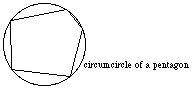index: click on a letter A B C D E F G H I J K L M N O P Q R S T U V W X Y Z A to Z index index: subject areas numbers & symbols sets, logic, proofs geometry algebra trigonometry advanced algebra & pre-calculus calculus advanced topics probability & statistics real world applications multimedia entrieswww.mathwords.com about mathwords website feedback

 Circumcircle Circumscribed Circle A circle that passes through all vertices of a plane figure and contains the entire figure in its interior. Note: All triangles have circumcircles and so do all regular polygons. Most other polygons do not.See also